We've recently introduced some new functionality in Chebfun for computing the nullspace of differential operators. Let's explore this with a couple of simple examples.

### 1. Simple example #1

Let's start as simply as we can, and take

$$(Lu)(x) := u''(x), \quad x\in [-1, 1].$$

L = chebop(@(u) diff(u, 2));

Clearly the nullspace of this operator -- that is, the space of functions $v$ for which $L(v)=0$ -- is spanned the two functions

v = [1, chebfun('x')];
norm(L(v))
ans =
0


Supposing we didn't know this, we could compute a basis for the nullspace with the null method:

LW = 'LineWidth'; lw = 1.6;
V = null(L)
plot(V, LW, lw)
V'*V
norm(L(V))
V =
chebfun column1 (1 smooth piece)
interval       length   endpoint values
[      -1,       1]        4      -1.4     0.44
Epslevel = 4.009500e-16.  Vscale = 1.384490e+00.
chebfun column2 (1 smooth piece)
interval       length   endpoint values
[      -1,       1]        4     -0.29      1.3
Epslevel = 4.132711e-16.  Vscale = 1.343214e+00.
ans =
1.000000000000000  -0.000000000000000
-0.000000000000000   1.000000000000000
ans =
2.644251170154956e-13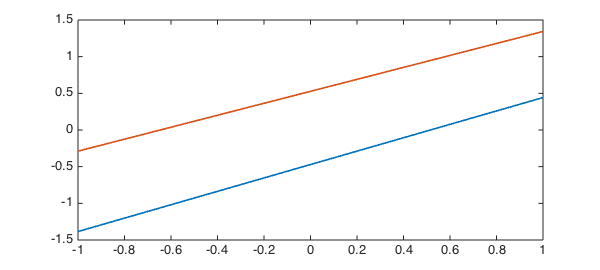where we find that $V^T V = I$ and $LV \approx 0$ as required.

Clearly V doesn't correspond directly to $1$ and $x$, since there is some freedom in how we orthogonalise the basis. However, we can check that V and ${1, x}$ correspond to the same spaces by computing the angle between the spaces with the subspace command.

subspace(v, V)
ans =
1.385544576431027e-14


### 2. Incomplete boundary conditions

Now let's consider the more complicated 2nd-order operator

$$Lu = u'' + 0.1x(1-x^2)u' + \sin(x)u, \quad x\in [-\pi, \pi]. \qquad (*)$$

dom = [-pi, pi];
L = chebop(@(x, u) diff(u, 2) + .1*x.*(1-x.^2).*diff(u) + sin(x).*u, dom);

As before, it has a nullspace of rank 2.

V = null(L)
plot(V, LW, lw)
V'*V
norm(L(V))
V =
chebfun column1 (1 smooth piece)
interval       length   endpoint values
[    -3.1,     3.1]       64       1.8    0.043
Epslevel = 1.753455e-16.  Vscale = 1.786120e+00.
chebfun column2 (1 smooth piece)
interval       length   endpoint values
[    -3.1,     3.1]       64    -0.011      1.3
Epslevel = 2.468482e-16.  Vscale = 1.268748e+00.
ans =
0.999999999999998   0.000000000000000
0.000000000000000   1.000000000000000
ans =
3.096261776816828e-11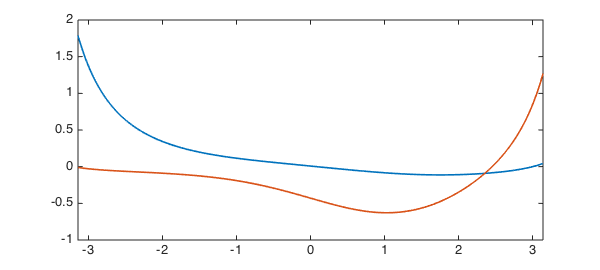However, now suppose we impose one boundary condition, say, a Dirichlet condition at the left. This removes one degree of freedom, and we are left with a rank 1 nullspace.

L.lbc = 0;
L.rbc = [];
v = null(L)
plot(v, LW, lw), shg
v'*v
norm(L(v))
v =
chebfun column (1 smooth piece)
interval       length   endpoint values
[    -3.1,     3.1]       64  -1.7e-16      1.3
Epslevel = 1.708133e-15.  Vscale = 1.268983e+00.
ans =
1.000000000000000
ans =
1.427155766422003e-11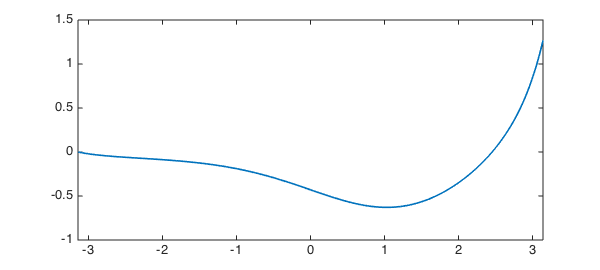Clearly this null vector must satisfy the given condition $v(-\pi) = 0.$

v(-pi)
ans =
-1.665334536937735e-16


### 3. An application

Where might these ideas be useful? Well, suppose we were interested in equation $(*)$ with a homogeneous Dirichlet condition at the left, and wanted to know what inhomogeneous Dirichlet condition gave the minimal 2-norm of the solution to $Lu = 1$. Rather than solving the linear system for a number of different boundary conditions (which would be computationally expensive) we could simply solve for one, say again a homogeneous Dirichlet condition,

L.rbc = 0;
u = L\1;
hold on, plot(u, '--r', LW, lw), hold off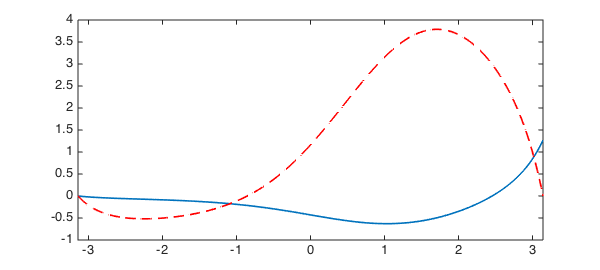and compute the rest by adding a scalar multiple of the null-function $v$.

E = chebfun(@(c) norm(u + c*v, 2), [-10, 10], 'vectorize', 'splitting', 'on');
plot(E,LW,lw)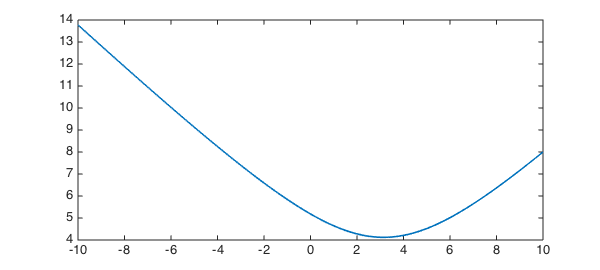We compute the 2-norm as a chebfun in the unknown variable $c$, which we can then minimise to obtain the minimal energy solution

[minE, c_star] = min(E)
u_star = u + c_star*v
plot(u_star,LW,lw)
minE =
4.121950420615883
c_star =
3.143771420957320
u_star =
chebfun column (1 smooth piece)
interval       length   endpoint values
[    -3.1,     3.1]       64    -4e-15        4
Epslevel = 1.776357e-15.  Vscale = 3.989391e+00.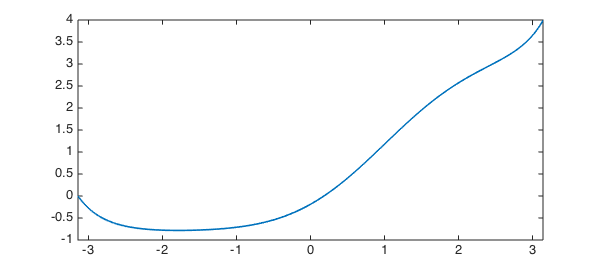So the condition we require is that $u(\pi)$ = bc_star, where

bc_star = u_star(pi)
bc_star =
3.989391428267542


### 4. Exotic constraints

The Chebfun null function can also handle the more exotic types of boundary conditions that can be imposed in Chebfun (see ). For example, suppose we wish to compute the nullspace of the 3rd-order piecewise-smoooth ODE

$$Lu := 0.1u''' + \sin(x)u'' + u, \quad x\in[-1,1]$$

with the 'boundary' condition

$$\int(u) = u(0).$$

dom = [-1, 1];
L = chebop(@(x, u) .1*diff(u, 3) + sin(x).*diff(u, 2) + u, dom);
L.bc = @(x, u) sum(u) - u(0);

Here null has no problems!

V = null(L)
plot(V, LW, lw), shg
V'*V
V =
chebfun column1 (1 smooth piece)
interval       length   endpoint values
[      -1,       1]       64       1.1     -1.2
Epslevel = 2.269192e-16.  Vscale = 1.223148e+00.
chebfun column2 (1 smooth piece)
interval       length   endpoint values
[      -1,       1]       64      0.77    -0.15
Epslevel = 2.567754e-16.  Vscale = 1.080928e+00.
ans =
1.000000000000000  -0.000000000000000
-0.000000000000000   1.000000000000001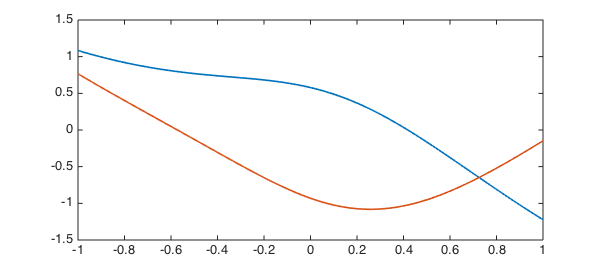sum(V) - V(0,:)
norm(L(V), 1)
ans =
1.0e-15 *
-0.111022302462516   0.666133814775094
ans =
9.372923955371351e-09


### 5. References

1. Chebfun Example ode-linear/NonstandardBCs